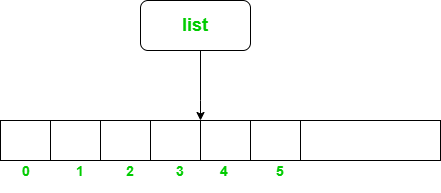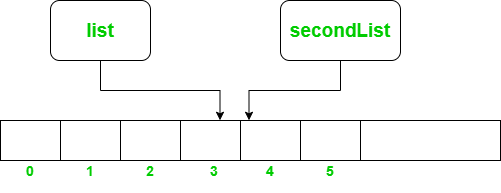Related Articles

# Can Two Variables Refer to the Same ArrayList in Java?

• Last Updated : 07 Jan, 2021

ArrayList class in Java is basically a resize-able array i.e. it can grow and shrink in size dynamically according to the values that we add to it. It is present in java.util package.

Syntax:

`ArrayList<E> list = new ArrayList<>();`

An ArrayList in java can be instantiated once with the help of the ‘new’ keyword and can be referred to by various objects/variables.The figure above shows how the object ‘list’ points to the ArrayList inside the memory. Now let us see how we can make another object to point to the same ArrayList that is instantiated above.

Syntax: Two variables refer to the same ArrayList:

`ArrayList<Integer> secondList = list;`Now when assigned the ‘list’ to the ‘secondList’ then the ‘secondList’ also starts pointing to the same ArrayList inside the memory. Notice here simply create a new instance. Now, for a better understanding let’s dive into code and see a few examples.

Below is the implementation of the problem statement:

## Java

 `// Java program to show how two objects can``// refer to same ArrayList in Java`` ` `import` `java.util.ArrayList;`` ` `public` `class` `GFG {``    ``public` `static` `void` `main(String[] args)``    ``{`` ` `        ``// Creating an ArrayList of Integer type in Java``        ``ArrayList list = ``new` `ArrayList<>();`` ` `        ``// Creating a new ArrayList object and making it``        ``// refer to the first ArrayList(list)``        ``ArrayList secondList = list;`` ` `        ``// Inserting some Integer values to the``        ``// arrayList using list variable/object``        ``list.add(``17``);``        ``list.add(``10``);``        ``list.add(``1``);``        ``list.add(``33``);``        ``list.add(``2``);`` ` `        ``// ArrayList after insertions : [17, 10, 1, 33, 2]`` ` `        ``// Displaying the ArrayList``        ``System.out.print(``"ArrayList after insertions: "``);``        ``display(list);`` ` `        ``// Modifying the ArrayList using secondList`` ` `        ``// Removing element from index 0``        ``secondList.remove(``0``);`` ` `        ``// Inserting Integer variables``        ``secondList.add(``51``);``        ``secondList.add(``99``);`` ` `        ``// ArrayList after modifications using``        ``// secondList: [10, 1, 33, 2, 51, 99]`` ` `        ``// Displaying the ArrayList``        ``System.out.print(``            ``"ArrayList after modifications using secondList: "``);``        ``display(list);``    ``}`` ` `    ``// Function to display an ArrayList``    ``public` `static` `void` `display(ArrayList list)``    ``{``        ``for` `(``int` `i = ``0``; i < list.size(); i++) {``            ``System.out.print(list.get(i) + ``" "``);``        ``}``        ``System.out.println();``    ``}``}`
Output
```ArrayList after insertions: 17 10 1 33 2
ArrayList after modifications using secondList: 10 1 33 2 51 99```

Attention reader! Don’t stop learning now. Get hold of all the important Java Foundation and Collections concepts with the Fundamentals of Java and Java Collections Course at a student-friendly price and become industry ready. To complete your preparation from learning a language to DS Algo and many more,  please refer Complete Interview Preparation Course.

My Personal Notes arrow_drop_up